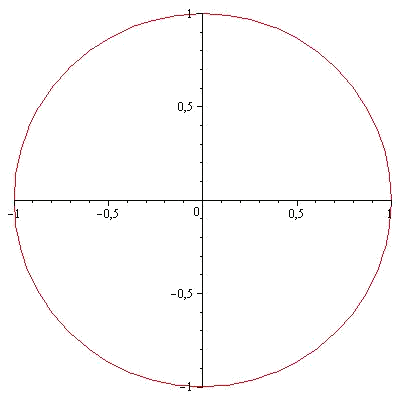# Areas of enclosures in the plane

We are going to introduce the calculation of the area in a region in the plane. If we call the region in the plane $$D$$, then:

$$\text{Area}(D)=\int_D 1 \ dx \ dy$$$This is a double integral. Therefore, the only thing we have to do is to obtain the parametric form of the region $$D$$ to be able to integrate it. As happens in calculation of integrals, sometimes it turns out to be easier to do a change of variables, the most typical being polar coordinates, to be able to calculate the area. In such a case, let's remember that we have to multiply the function that we integrate by the determinant of the Jacobian matrix of the change of variable that we are using. We are now going to calculate the area of a circle with radius $$R$$. We will suppose that it is centered on the origin, so that it is given by the equation: $$x^2+y^2\leqslant R^2$$.This region one can be written in parametric components using polar coordinates: $$\left\{ \begin{array}{l} x=r\cos(\theta) \\ y=r \sin(\theta) \end{array} \right. \quad \text{ with } \quad r\in[0,R] \ \text{ and } \ \theta\in[0,2\pi]$$$

We must remember that when doing a change of variables we must multiply the function to be integrated by the determinant of the Jacobian matrix, which in this case is $$r$$. Then:

$$\int_0^R \int_0^{2\pi} 1\cdot r \ d\theta \ dr$$\$